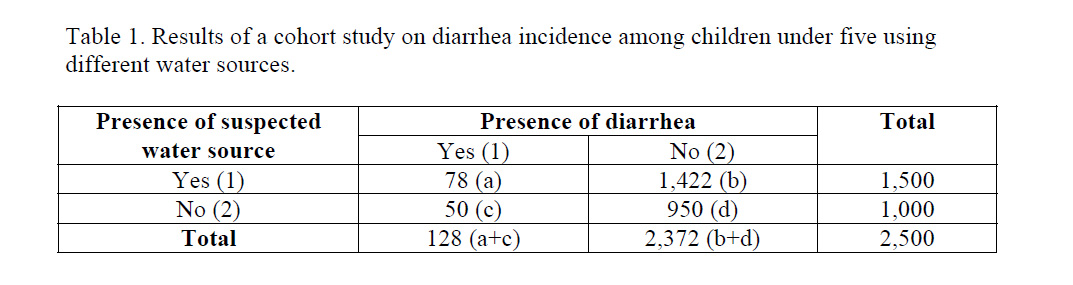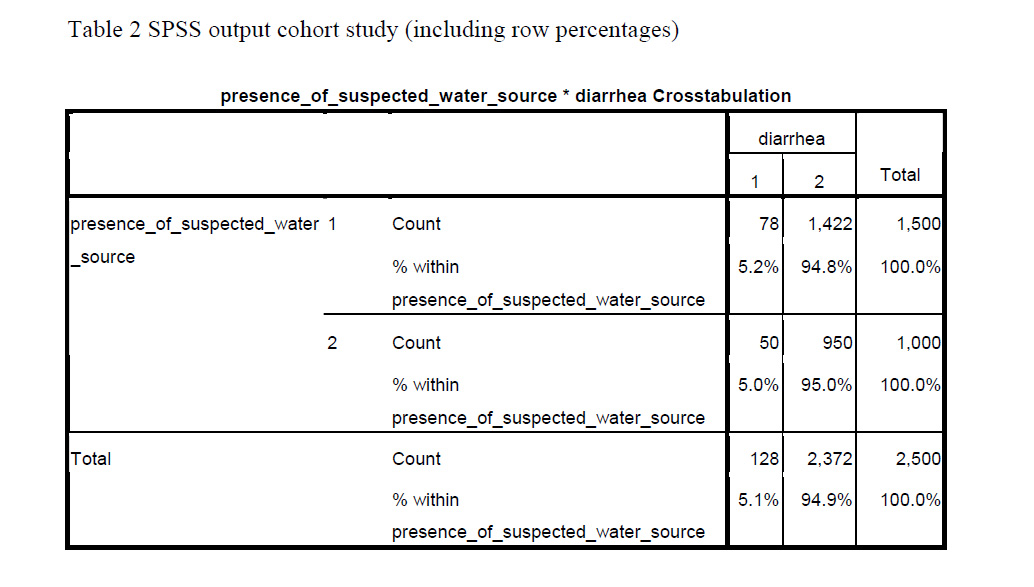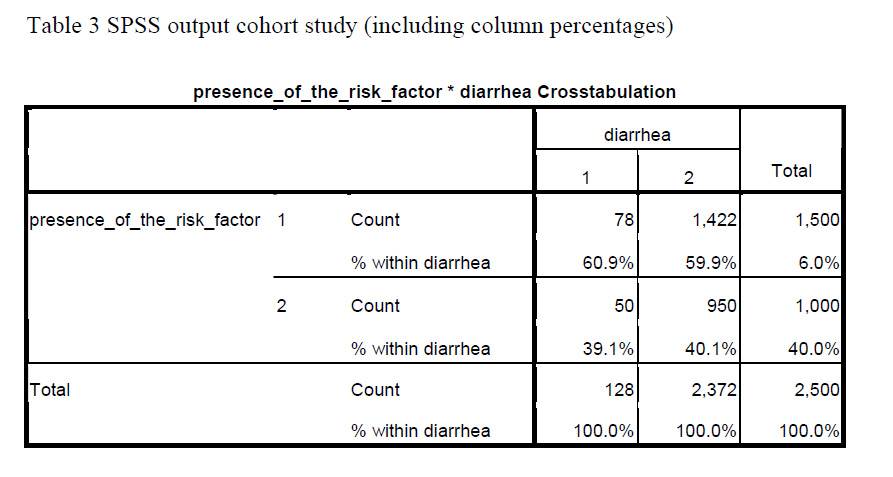# Crosstabulation

### Effect Size Statistics: How to Calculate the Odds Ratio from a Chi-Square Cross-tabulation Table

August 12th, 2020 by

Lest you believe that odds ratios are merely the domain of logistic regression, I’m here to tell you it’s not true.

One of the simplest ways to calculate an odds ratio is from a cross tabulation table.

We usually analyze these tables with a categorical statistical test. There are a few options, depending on the sample size and the design, but common ones are Chi-Square test of independence or homogeneity, or a Fisher’s exact test.

### Cross-tabulation in Cohort and Case-Control Studies

September 3rd, 2010 by

by Annette Gerritsen, Ph.D.

### Cross-tabulation in cohort studies

Assume you have just done a cohort study. How do you actually do the cross-tabulation to calculate the cumulative incidence in both groups?

Best is to always put the outcome variable (disease yes/no) in the columns and the exposure variable in the rows. In other words, put the dependent variable–the one that describes the problem under study–in the columns. And put the independent variable–the factor assumed to cause the problem–in the rows.

Let’s take as example a cohort study used to see whether there is a causal relationship between the use of a certain water source and the incidence of diarrhea among children under five in a village with different water sources. In this case, the variable diarrhea (yes/no) should be in the columns. The variable water source (suspected/other) should be in the rows.

SPSS will put the lowest value of the variable in the first column or row. So in order to get those with diarrhea in the first column you should label ‘diarrhea’ as 1 and ‘no diarrhea’ as 2. The same is true for the exposure variable: label the ‘suspected water source’ as 1 and the ‘other water source’ as 2.

You will then be able to calculate the cumulative incidence (risk of developing the disease) among those with the exposure: a / (a + b) and among those without the exposure: c / (c + d).

In the case of the diarrhea study (Table 1), you could calculate the cumulative incidence of diarrhea among those exposed to the suspected water source, which would be (78 / 1,500 =) 5.2%.

You can also do this for those exposed to other water sources, which would be (50 / 1,000 =) 5.0%.SPSS can give you these percentages immediately (in cell ‘a’ and ‘c’ respectively), when you ask to display row percentages in the Cells option (Table 2).### Cross-tabulation in Case-Control Studies

When you have used a case-control design for the diarrhea study, the actual cross-tabulation is quite similar, only “presence of diarrhea yes/no”, is now changed into “cases” and “controls.

Label the cases as 1, and the controls as 2. Be aware that row percentages have no meaning in terms of occurrence of disease in case-control studies. This is because in case-control studies the researcher determines how many patients and how many controls are included.

The ratio between the number of patients and controls (e.g. 2 : 1 or 4 : 1) influences the row percentages. So in a case-control study, the cumulative incidence cannot be calculated.

When having conducted a case-control study, you can ask to display column percentages. That gives you the proportion of those exposed to the suspected water source among the cases (in cell ‘a’) and among the controls (in cell ‘b’).

Table 3 gives the SPSS output for the same diarrhea study assuming that it had a case-control design. Using the data provided, (78 / 128 =) 60.9% of the cases were exposed to the suspected water source, while this was (1,422 / 2,372 =) 59.9% of the controls (asked for column percentages).Another article will be devoted to measures of association: How do you actually compare cumulative incidence rates in cohort studies? And what measure of association can be used in case-control studies?

About the Author: With expertise in epidemiology, biostatistics and quantitative research projects, Annette Gerritsen, Ph.D. provides services to her clients focussing on the methodological soundness of each phase of an epidemiological study to ensure getting valid answers to the proposed research questions. She is the founder of Epi Result.